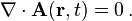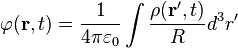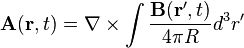# Understanding Magnetic Field in Length Gauge

• DrClaude

#### DrClaude

Mentor
I've having trouble understanding one of the consequences of using the length gauge.

The length gauge is obtained by the gauge transformation ##\mathbf{A} \rightarrow \mathbf{A} + \nabla \chi## with ##\chi = - \mathbf{r} \cdot \mathbf{A}##. Starting from the Coulomb gauge, we have
\begin{align*} \mathbf{A}_\mathrm{L} &= \mathbf{A}_\mathrm{C} + \nabla( -\mathbf{r} \cdot \mathbf{A}_\mathrm{C} ) \\ &= \mathbf{A}_\mathrm{C} - \mathbf{A}_\mathrm{C} = 0 \end{align*}
How can I reconcile this with ##\mathbf{B} = \nabla \times \mathbf{A}##? It appears that there can be no magnetic field in the length gauge, while the gauge transformation leaves the magnetic field unchanged...

The Coulomb gauge (also known as the transverse gauge) is much used in physics and is defined by the gauge condition (more precisely, gauge fixing condition)It is particularly useful for "semi-classical" calculations in quantum mechanics, in which the vector potential is quantized but the Coulomb interaction is not.

The Coulomb gauge has a number of properties:

1. The potentials can be expressed in terms of instantaneous values of the fields and densities (in SI units)where ρ(r, t) is the electric charge density,...

2. so i will request you to visit wiki and see how your length gauge is related to coulomb vector potential/or see griffiths book on electrodynamics-

Thanks for your reply, but unfortunately there is nothing in it that I didn't already know. I am simply concerned about the consequences of the vector potential vanishing in the length gauge.

This might be a stupid question, but why do you expect this?$$\nabla( -\mathbf{r} \cdot \mathbf{A}_\mathrm{C} ) = - \mathbf{A}_\mathrm{C}$$
Consider ##\mathbf{A}_\mathrm{C} = \mathbf{f} \times \mathbf{r}## for some vector f. The product with r will vanish.

•drvrm
Do you know tensor calculus? If so, you need to compute ## \partial_{i} \left(-x_j A_{C,j}\right) ##.

This might be a stupid question, but why do you expect this?$$\nabla( -\mathbf{r} \cdot \mathbf{A}_\mathrm{C} ) = - \mathbf{A}_\mathrm{C}$$
That's I "known result" that I never bothered to rederive. I'll have to add that to my list of things-to-do.

I've been doing some reading, and I think I now understand what is going on.

First, some background (that should've been present in the OP). In non-relativistic QM, when considering the interaction of charged particles with electromagnetic radiation, it is common to use the Coulomb gauge. But when considering the interaction of atoms and molecules with intense laser fields, other gauges are commonly used, such as the acceleration gauge or the length gauge.

I always understood these gauge transformations to have no influence on the physics, and it appears that this is incorrect. Things like transition amplitudes can be different depending on the gauge chosen. Also, these certain gauges have limited applicability, and this appears to be the case here. In the length gauge, ##\mathbf{B} = 0##. Working in the length gauge is equivalent to making the dipole approximation (or long-wavelength approximation), and is only applicable in the same parameter range as the latter. While I understand the limitations related to the dipole approximation, these limitations were hidden to me when looking at the length gauge.

For those who would like to know more, I recommend the following set of slides. No author is given, but it is most probably the same author as http://arxiv.org/abs/1304.2303

I definitely will have learned something new today!

•Greg Bernhardt
The Coulomb gauge (also known as the transverse gauge) is much used in physics and is defined by the gauge condition (more precisely, gauge fixing condition)It is particularly useful for "semi-classical" calculations in quantum mechanics, in which the vector potential is quantized but the Coulomb interaction is not.

The Coulomb gauge has a number of properties:

1. The potentials can be expressed in terms of instantaneous values of the fields and densities (in SI units)where ρ(r, t) is the electric charge density,...

2. so i will request you to visit wiki and see how your length gauge is related to coulomb vector potential/or see griffiths book on electrodynamics-
First of all, let's get this straight, because it's obviously not correct. The vector potential is not simply instantaneous (this cannot be, because relativity forbids the observable quantities, i.e., the electromagnetic field to relate in an action-at-a-distance way with the sources, i.e., the current and charge densities).

\begin{equation}
\label{1}
F_{\mu \nu}=\partial_{\mu} A_{\nu} - \partial_{\nu} A_{\mu},
\end{equation}
which uses the homogeneous Maxwell equations, which are constraint equations on the electromagnetic field. So we can forget about them completely with this ansatz with the four-vector potential. The disadvantage is that the four-potential is not observable, because it is not completely determined by the dynamical equations of motion, which come from the inhomogeneous Maxwell equations, which read
\begin{equation}
\label{2}
\partial_{\mu} F^{\mu \nu} = \frac{1}{c} j^{\nu}.
\end{equation}
The reason is "gauge invariance". Indeed the field-strength tensor does not change, when we add an arbitrary four-gradient field to the four-potential, i.e.,
\begin{equation}
\label{3}
A_{\mu}'=A_{\mu}+\partial_{\mu} \chi.
\end{equation}
Thus, we have to constrain the four-potential by one additional condition, which at least partially "fixes the gauge", so that the resulting dynamical equations have unique solutions.

Common gauges are the Lorenz gauge, which has the advantage of being manifestly covariant or the Coulomb gauge, which has advanatages in some calculations and in quantizing the electromagnetic field, but the disadvantage of spoiling manifest Lorentz covariance. A naive Lorentz transformation usually leads to four-potentials in another gauge, if the gauge condition is not a four-tensor equation. Of course, the general Lorentz transformation of the four-potential is a naive Lorentz transformation of the four-potential as a vector field followed by an arbitrary gauge transformation. Expressed in the new coordinates, you can restore the chosen gauge also to be valid in the new frame, but that's not relevant here. We just work in a fixed inertial reference frame.

\begin{equation}
\label{4}
\vec{\nabla} \cdot \vec{A}=0.
\end{equation}
Because this is not Lorentz covariant, it is simpler to use the 1+3-dimensional formalism. The fields read
\begin{equation}
\label{5}
\vec{E}=-\frac{1}{c} \partial_t \vec{A} -\vec{\nabla} \Phi, \quad \vec{B}=\vec{\nabla} \times \vec{A}.
\end{equation}
I've written ##A^0=\Phi##. The gauge transformation (\ref{3}) translates into
\begin{equation}
\label{6}
\Phi'=\Phi+\frac{1}{c} \partial_t \chi, \quad \vec{A}'=\vec{A}-\vec{\nabla} \chi.
\end{equation}
\begin{equation}
\label{7}
\vec{\nabla} \times \vec{B}-\frac{1}{c} \partial_t \vec{E}=\frac{1}{c} \vec{j}, \quad \vec{\nabla} \cdot \vec{E}=\rho.
\end{equation}
Plugging in (\ref{5}) we get
$$\vec{\nabla} \times (\vec{\nabla} \times \vec{A}) + \frac{1}{c} \partial_t \left (\frac{1}{c} \partial_t \vec{A}+\vec{\nabla} \Phi \right )=\frac{1}{c} \vec{j}$$
and with the Coulomb gauge condition (\ref{4})
\begin{equation}
\label{8}
\Box \vec{A}=\frac{1}{c} (\vec{j}-\vec{\nabla} \partial_t \Phi)=\frac{1}{c} \vec{j}_{\perp}.
\end{equation}
Further we have
\begin{equation}
\label{9}
\vec{\nabla} \cdot \vec{E}=-\vec{\nabla} \left (\frac{1}{c} \vec{A}+\vec{\nabla} \Phi \right)=-\Delta \Phi=\rho.
\end{equation}
Thus we have to use the retarded propagator to solve (\ref{8}),
\begin{equation}
\label{10}
\vec{A}(t,\vec{x})=\int_{\mathbb{R}^3} \mathrm{d}^3 \vec{x}' \frac{\vec{j}_{\perp}(t-|\vec{x}-\vec{x}'|/c,\vec{x}')}{4 \pi c |\vec{x}-\vec{x}'|}.
\end{equation}
For the scalar potential we get from (\ref{9})
\begin{equation}
\label{11}
\Phi(t,\vec{x})=\int_{\mathbb{R}^3} \mathrm{d}^3 \vec{x}' \frac{\rho(t,\vec{x})}{4 \pi |\vec{x}-\vec{x}'|},
\end{equation}
which appears not to be causal. One can, however prove, that the fields ##(\vec{E},\vec{B})## from the found solution for the four-potential is a retraded solution with the physical sources ##\rho## and ##\vec{j}## (Jefimenko equations).

Of course, we must have charge conservation, which is a integrability constraint, resulting from gauge invariance,
\begin{equation}
\label{12}
\partial_t \rho + \vec{\nabla} \cdot \vec{j}=0,
\end{equation}
and we thus have
\begin{equation}
\label{13}
\vec{\nabla} \cdot \vec{j}_{\perp}=\vec{\nabla} \cdot \vec{j}-\partial_t \Delta \Phi=0,
\end{equation}
because of (\ref{9}).

Finally to the original question. I don't understand it at all, because, of course, just using
##\chi=\vec{x} \cdot \vec{A}##
in the gauge transformation (\ref{6}) yields for the magnetic field
$$\vec{A}'=\vec{A}-\vec{\nabla} (\vec{x} \cdot \vec{A}).$$
Now writing this in the Ricci calculus (in 3D Cartesian coordinates, where I write all vector indices as lower indices) we get
$$A_j'=A_j-\partial_j (x_k A_k)=-x_k \partial_j A_k \neq 0.$$

•jim mcnamara
We don't need to burden ourselves with electrodynamics to get at the meat of this question.

Basically, given a vector field ##\bf{A}##, we make a (gauge) transformation ##\bf{A}'=\bf{A}+\nabla\phi##. Due to the nature of the curl operator, we can see that the curl of the two vector fields must be equal: ##\bf{B}=\nabla\times\bf{A}=\nabla\times\bf{A}'##. This is just math. Physics can not refute this result. No matter what, changing a gauge condition can not change the curl. As such, there is no way that by changing a gauge you can "gauge away" a magnetic field. If a magnetic field is present in any gauge, that same magnetic field is present in every other gauge. If this statement is wrong, then I have misunderstood basically all of electrodynamics! (I certainly hope that's not the case).

Now, in your original formula you have these lines: ##\bf{A}_L=\bf{A}_C+\nabla(-\bf{r}\cdot\bf{A}_C)## and then ##\bf{A}_L=\bf{A}_C-\bf{A}_C=0##

Concentrate on the second line. What have you done? You have made the equality ##\bf{A}_C=\nabla({\bf{r}\cdot\bf{A}_C})##. In other words, you have set ##\bf{A}_C## to equal the gradient of some scalar function. Since your Coulomb gauge vector potential can be expressed as the gradient of a scalar function, it must necessarily be curl-free. In other words, only Coulomb gauge vector potentials which give rise to 0 magnetic field can be expressed in this way. So what you have really made the statement of is transforming from one gauge where the magnetic field is 0 to another gauge where the magnetic field is also (identically) 0. This is not a paradox.

So I would go back to what mfb said. Why do you expect ##\bf{A}_C=\nabla({\bf{r}\cdot\bf{A}_C})## to hold? This equation should only be able to work under the specific circumstance that ##\bf{A}_C## is curl free.

•mfb and DrClaude
As I already calculated some time ago, the original calculation is a simple mistake. The correct regauged potential is
$$A_j'=A_j-\partial_j(x_k A_k)=A_j-\delta_{jk}A_k-x_k \partial_j A_k=-x_k \partial_j A_k.$$
Of course the curl and thus the magnetic field is the same in both gauges, because
$$(\vec{\nabla} \times \vec{A}')_a=\epsilon_{abc} \partial_b A_c'=-\epsilon_{abc} \partial_b x_k \partial_c A_k=-\epsilon_{abc} (\delta_{bk} \partial_c A_k+x_k \partial_b \partial_c A_k)=-\epsilon_{abc} \partial_c A_b=+\epsilon_{acb} \partial_c A_b =(\vec{\nabla} \times \vec{A})_a.$$
As Matterwave said, this must be so, because regauging the vector potential doesn't change its curl, because ##\vec{\nabla} \times \vec{\nabla}\chi=0## for any scalar field ##\chi##. That's what's behind the entire gauge business to begin with!